Next: Uncertainty Relation Up: Fundamental Concepts Previous: Degeneracy

# Compatible Observables

Suppose that we wish to simultaneously measure two observables,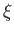and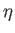, of a microscopic system. Let us assume that we possess an apparatus that is capable of measuring, and another that can measure. For instance, the two observables in question might be the projection in the- and-directions of the spin angular momentum of a spin one-half particle. These could be measured using appropriate Stern-Gerlach apparatuses . Suppose that we make a measurement of, and the system is consequently thrown into one of the eigenstates of,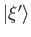, with eigenvalue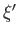. What happens if we now make a measurement of? Well, suppose that the eigenstateis also an eigenstate of, with eigenvalue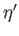. In this case, a measurement ofwill definitely give the result. A second measurement ofwill definitely give the result, and so on. In this sense, we can say that the observablesandsimultaneously have the valuesand, respectively. Clearly, if all eigenstates ofare also eigenstates ofthen it is always possible to make a simultaneous measurement ofand. Such observables are termed compatible.

Suppose, however, that the eigenstates ofare not eigenstates of. Is it still possible to measure both observables simultaneously? Let us again make an observation ofthat throws the system into an eigenstate, with eigenvalue. We can now make a second observation to determine. This will throw the system into one of the (many) eigenstates ofthat depend on. In principle, each of these eigenstates is associated with a different result of the measurement. Suppose that the system is thrown into an eigenstate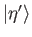, with the eigenvalue. Another measurement ofwill throw the system into one of the (many) eigenstates ofthat depend on. Each eigenstate is again associated with a different possible result of the measurement. It is clear that if the observablesanddo not possess simultaneous eigenstates then if the value ofis known (i.e., the system is in an eigenstate of) then the value ofis uncertain (i.e., the system is not in an eigenstate of), and vice versa. We say that the two observables are incompatible.

We have seen that the condition for two observablesandto be simultaneously measurable is that they should possess simultaneous eigenstates (i.e., every eigenstate ofshould also be an eigenstate of). Suppose that this is the case. Let a general eigenstate of, with eigenvalue, also be an eigenstate of, with eigenvalue. It is convenient to denote this simultaneous eigenstate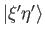. We have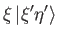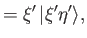(1.66)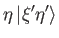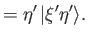(1.67)

We can left-multiply the first equation by, and the second equation by, and then take the difference. The result is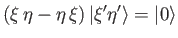(1.68)

for each simultaneous eigenstate. Recall that the eigenstates of an observable must form a complete set. It follows that the simultaneous eigenstates of two observables must also form a complete set. Thus, the previous equation implies that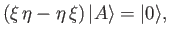(1.69)

where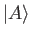is a general ket. The only way that this can be true is if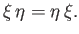(1.70)

We conclude that the condition for two observablesandto be simultaneously measurable is that they should commute.Next: Uncertainty Relation Up: Fundamental Concepts Previous: Degeneracy
Richard Fitzpatrick 2016-01-22